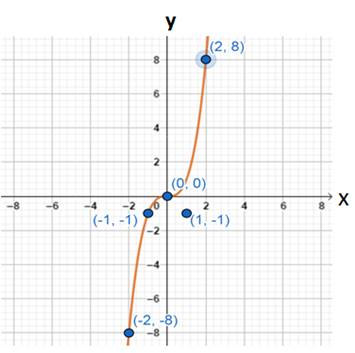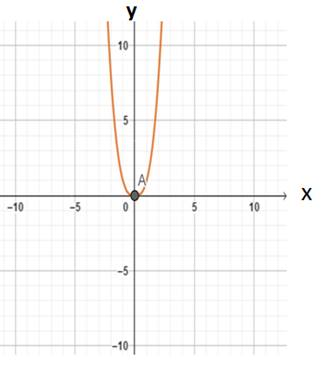# To sketch the graph of function### Precalculus: Mathematics for Calcu...

6th Edition
Stewart + 5 others
Publisher: Cengage Learning
ISBN: 9780840068071### Precalculus: Mathematics for Calcu...

6th Edition
Stewart + 5 others
Publisher: Cengage Learning
ISBN: 9780840068071

#### Solutions

Chapter 2.5, Problem 88E

a.

To determine

## To sketch the graph of function

Expert Solution

### Explanation of Solution

Given information : The function is f(x)=x3

Graph : This function by plotting points

Make a table of values of the function for several values of x

 x -2 -1 0 1 2 x3 -8 -1 0 1 8Interpretation : The above graph of function f(x)=x3

From the graph the points are

(2,8)(0,0)(2,8)(1,1)(1,1)

b.

To determine

Expert Solution

### Explanation of Solution

Given information : The function is g(x)=|x3|

Graph : This function by plotting points

Make a table of values of the function for several values of x

 x -2 0 1 |x3| 8 0 1Interpretation : The above graph of function g(x)=|x3| .

### Have a homework question?

Subscribe to bartleby learn! Ask subject matter experts 30 homework questions each month. Plus, you’ll have access to millions of step-by-step textbook answers!